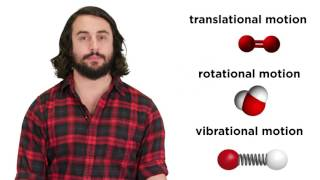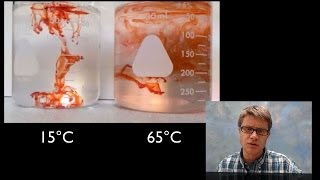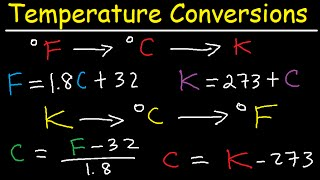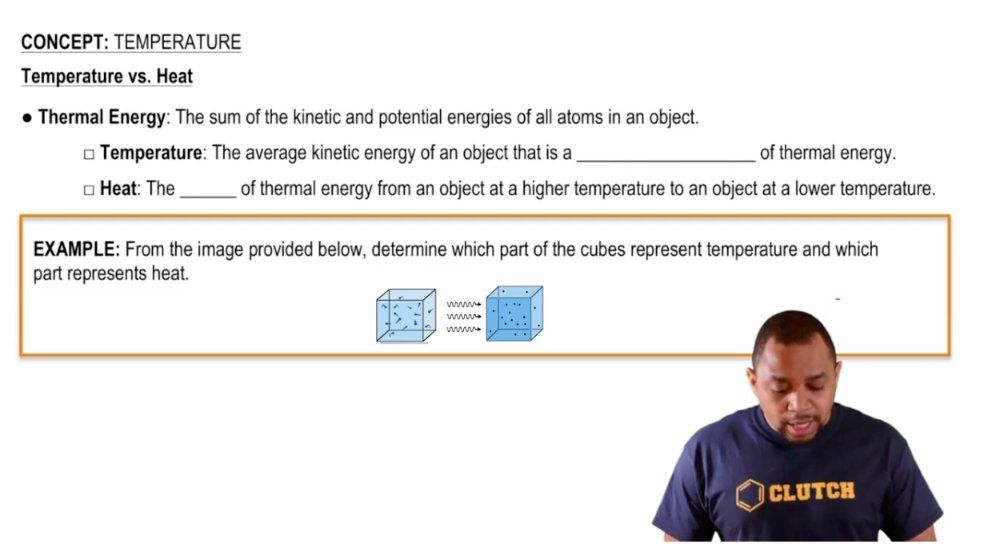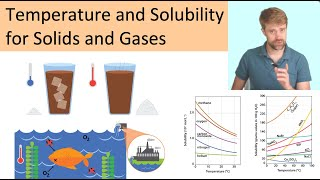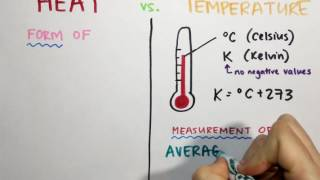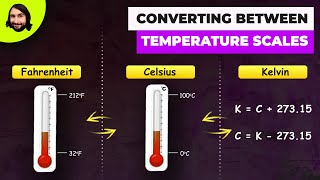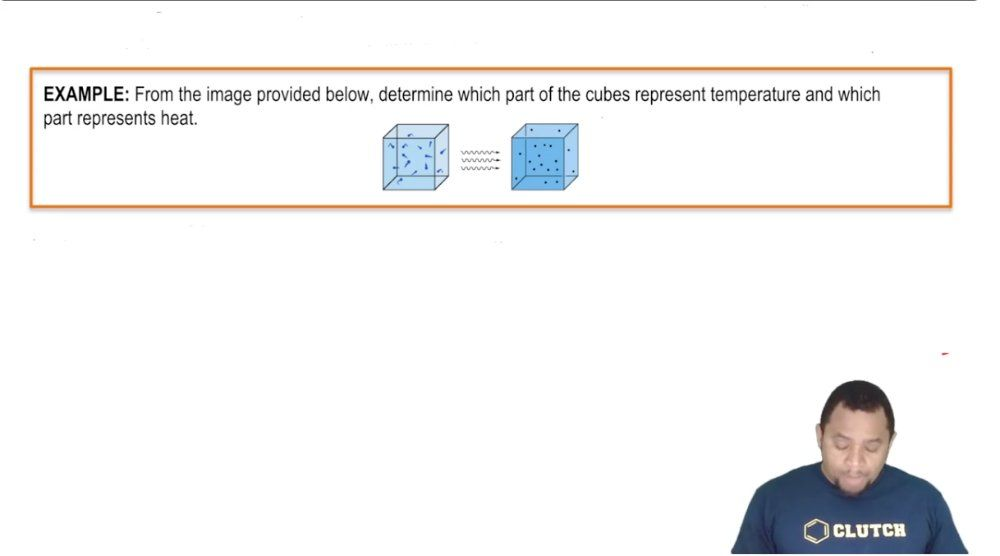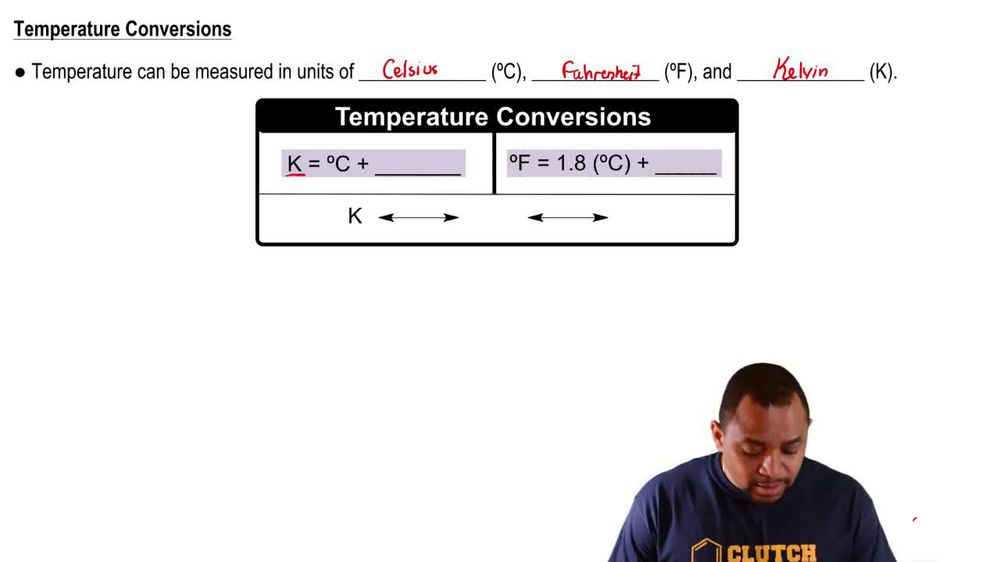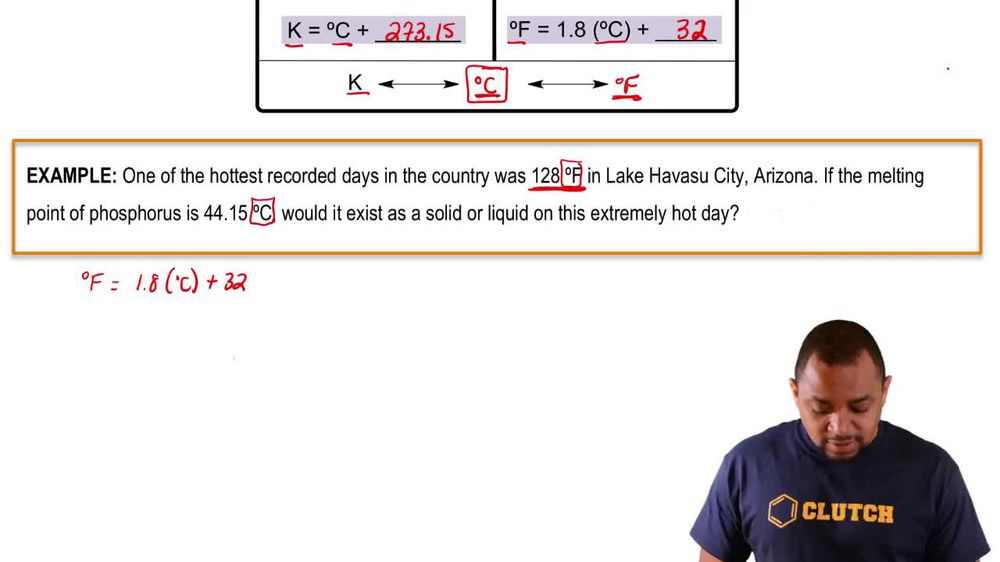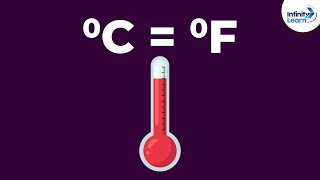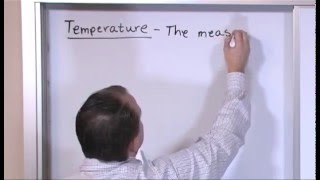Start typing, then use the up and down arrows to select an option from the list.
1. 1. Intro to General Chemistry2. Temperature# Temperature Example 1

by Jules Bruno
113 views
0
hey everyone. So here in this example question it says the image provided below determine which part of the cubes represent temperature and which part represents heat. So remember heat itself just represents the flow of thermal energy from an object that's hotter to an object that's colder. If we take a look here, we have these two cubes and we can see that we have a set of wavy lines between them. These set of wavy lines themselves represent our heat. Temperature itself is a result of the movement of molecules within a given structure. So if we take a look here, we can see that in the first cube, let's call it Cube one. We can see that we have these little balls that are moving around and in the second cube, cube two we have the same balls but they're not moving as vigorously. We're gonna say here temperature is really just the movement in each cube. So you could say that temperature is represented by the molecules moving in this cube as well as in this cube. Remember we said that heat is the flow of energy from a hotter object to a colder object. The objects in the first cube, we can see them moving more vigorously more more actively. Therefore they would generate higher temperature. So we'd say that Cube one would have a higher temperature And the balls in the 2nd Cube are moving slower. So they have a lower temperature. This reinforces the idea that heat which we said are these wavy lines would go from cuba one to cube to they're going from a higher temperature to a lower temperature. So keep this in mind when learning the distinction between heat and temperature.